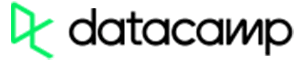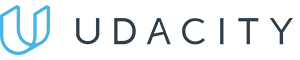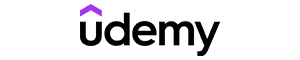Code has been added to clipboard!

# Main Tips on Using JavaScript Math Functions in a Nutshell

Published Aug 8, 2017
Updated Oct 1, 2019

JavaScript math Functions are built-in and static. They allow developers to implement not only basic mathematical operations but advanced calculations as well.

Working with JavaScript built-in math object can be highly useful, whether you want to build a calculator for your website or generate a random number for a guessing game. In this tutorial, you will learn to work with the JavaScript math object. You will learn all about its syntax, the most popular methods and properties.

## JavaScript Math Object: Main Tips

• This object allows mathematical tasks to be performed on numbers.
• The JavaScript Math object includes many methods and properties you can apply.
• This object has no constructor and cannot be created first.

## Math Functions

In this chapter, we will present you with the most commonly used math functions. Each of them will be explained in a short and clear manner for you to grasp each concept quickly. Code examples will be helpful, too. If you wish to read more on math functions, don't hesitate to visit this tutorial we have created for easy referral.

#### Math.max() and Math.min()

You can use `Math.max()` JavaScript to find the highest out of listed values, and `Math.min()` to find the lowest:

Example
``Math.min(65, 951, 84, 20, -98, -235);``

Example
``Math.max(65, 951, 84, 20, -98, -235);``

#### Math.random()

`Math.random()` will return a random numeric value between 0 (inclusive), and 1 (exclusive):

Example
``Math.random();``

#### Math.round()

JavaScript `Math.round()` is used to round a number to the nearest integer, up or down:

Example
``````Math.round(5.8);
Math.round(3.2);``````

#### Math.ceil()

`Math.ceil()` is used to round a number up to the nearest integer:

Example
``````Math.ceil(5.8);
Math.ceil(3.2);``````

#### Math.floor()

JavaScript `Math.floor()` is used to round a number down to the nearest integer:

Example
``````Math.floor(5.8);
Math.floor(3.2);``````

## Get a Random Number

You can use `Math.random()` and `Math.floor()` together to get a random integer ranging from 0 to 10.

In the example below, `Math.random()` generates a random decimal number between 0 and 1, which is multiplied by 10 to get a decimal number between 0 and 10. Then, `Math.floor()` method rounds that number down to an integer:

Example
``Math.floor(Math.random() * 10);``Pros
• Easy to use with a learn-by-doing approach
• Offers quality content
• Gamified in-browser coding experience
• The price matches the quality
• Suitable for learners ranging from beginner to advanced
Main Features
• Free certificates of completion
• Focused on data science skills
• Flexible learning timetablePros
• Simplistic design (no unnecessary information)
• High-quality courses (even the free ones)
• Variety of features
Main Features
• Nanodegree programs
• Suitable for enterprises
• Paid Certificates of completionPros
• Easy to navigate
• No technical issues
• Seems to care about its users
Main Features
• Huge variety of courses
• 30-day refund policy
• Free certificates of completion

## Properties for Constants

Math object properties are used for math constants. You are probably familiar with some of them, like the Pi number, or Euler's number. In cases when you need them in your JavaScript code, you might use the properties listed in the tables below. You will find both names of the constant and approximate values defined for each one.

Property Description
E Return Euler's number (approximately 2.718)
LN2 Return natural logarithm of 2 (approximately 0.693)
LN10 Return natural logarithm of 10 (approximately 2.302)
LOG2E Return base-2 logarithm of E (approximately 1.442)
LOG10E Return base-10 logarithm of E (approximately 0.434)
PI Return PI (approximately 3.1416)
SQRT1_2 Return square root of 1/2 (approximately 0.707)
SQRT2 Return square root of 2 (approximately 1.414)

## Methods To Use

You can use various methods for working with math objects, too. They will let you get arithmetic as well as trigonometric values. See the table below to get familiarized with them. As you learn to use these methods, working with math objects will be a breeze.

Method Description
abs(var1) Return absolute value of var1
acos(var1) Return arccosine of var1 in radians
asin(var1) Return arcsine of var1 in radians
atan(var1) Return arctangent of var1 as numeric value between -PI/2 and PI/2 radians
atan2(var2,var1) Return arctangent of the quotient of arguments
ceil(var1) Return var1 rounded upwards to nearest integer
cos(var1) Return cosine of var1 (var1 is in radians)
exp(var1) Return value of Evar1
floor(var1) Return var1 rounded downwards to nearest integer
log(var1) Return natural logarithm (base E) of var1
max(var1,var2,var3,...,n) Return number with highest value
min(var1,var2,var3,...,n) Return number with lowest value
pow(var1,var2) Return value of var1 to power of var2
random() Return random number between 0 and 1
round(var1) Round var1 to nearest integer
sin(var1) Return sine of var1 (var1 is in radians)
sqrt(var1) Return square root of var1
tan(var1) Return tangent of angle

## JavaScript Math Object: Summary

• `Math` object allows you to perform JavaScript math functions.
• The `Math` object has many methods and properties you can use.
• You can use `Math` object to get a random number.

Days
Hours
Minutes
Seconds